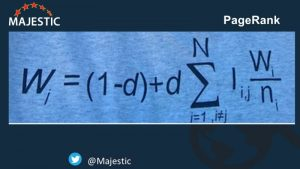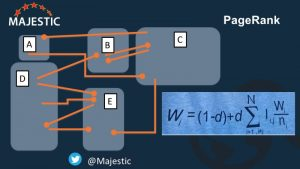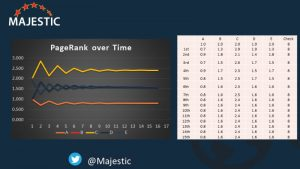Earlier today, Dixon Jones from Majestic shared on Twitter a thorough, digestible explanation of how PageRank actually works.

I gave it a watch myself, and thought it was a good moment to revisit this wild piece of math that has made quite a dent on the world over the past 20 years.

As a sidenote, we know as of 2017 that while PageRank was removed from the Toolbar in 2016, it still forms an important part of the overall ranking algorithm, and thus is worthwhile to understand.

Jones starts with the simple — or at least, straightforward — formula.For those who don’t adore math, or who may have forgotten a few technical terms since the last calculus class, this formula would be read aloud like this:

“The PageRank of a page in this iteration equals 1 minus a damping factor, plus, for every link into the page (except for links to itself), add the page rank of that page divided by the number of outbound links on the page and reduced by the damping factor.”

Back to the original Google paper

At this point, Jones moves forward in the video to a simpler, still useful version of the calculation. He pulls out excel, an easy 5 node visual, and maps out the ranking algorithm over 15 iterations. Great stuff.

Personally, I wanted a bit more of the math, so I went back and read the full-length version of “The Anatomy of a Large-Scale Hypertextual Web Search Engine” (a natural first step). This was the paper written by Larry Page and Sergey Brin in 1997. Aka the paper in which they presented Google, published in the Stanford Computer Science Department. (Yes, it is long and I will be working a bit late tonight. All in good fun!)

How’s this for an opening line: “In this paper, we present Google, a prototype of a large-scale search engine which makes heavy use of the structure present in hypertext.

Casual, per their overall, continuing style.

As an extra fun fact, our very own Search Engine Watch was cited in that Google debut paper! By none other than Page and Brin themselves, stating that there were already 100 million web documents as of November 1997.

Anyway, back to work.

Here’s how the PageRank calculation was originally defined:

“Academic citation literature has been applied to the web, largely by counting citations or backlinks to a given page. This gives some approximation of a page’s importance or quality. PageRank extends this idea by not counting links from all pages equally, and by normalizing by the number of links on a page. PageRank is defined as follows:

We assume page A has pages T1…Tn which point to it (i.e., are citations). The parameter d is a damping factor which can be set between 0 and 1. We usually set d to 0.85. There are more details about d in the next section. Also C(A) is defined as the number of links going out of page A. The PageRank of a page A is given as follows:

PR(A) = (1-d) + d (PR(T1)/C(T1) + … + PR(Tn)/C(Tn))

Note that the PageRanks form a probability distribution over web pages, so the sum of all web pages’ PageRanks will be one.

PageRank or PR(A) can be calculated using a simple iterative algorithm, and corresponds to the principal eigenvector of the normalized link matrix of the web. Also, a PageRank for 26 million web pages can be computed in a few hours on a medium size workstation. There are many other details which are beyond the scope of this paper.”

What does that mean?

Bear with us! Here’s our formula again:

PR(A) = (1-d) + d (PR(T1)/C(T1) + … + PR(Tn)/C(Tn))

Note this is the same as the picture above, except that the photo “simplifies” the second part of the equation by substituting an upper case sigma (∑), which is the symbol for a mathematical summation, i.e. do this formula for all pages 1 through n and then add them up.

So to calculate the PageRank of given page A, we first take 1 minus the damping factor (d). D is typically set as .85, as seen in their original paper.

We then take the PageRanks of all pages that point to and from page A, add them up, and multiply by the damping factor of 0.85.

Not that bad, right? Easier said than done.

PageRank is an iterative algorithm

Perhaps your eyes glazed over this part, but Brin and Sergey actually used the word “eigenvector” in their definition. I had to look it up.

Apparently, eigenvectors play a prominent role in differential equations. The prefix “eigen” comes from German for “proper” or “characteristic.” There also exist eigenvalues and eigenequations.

As Rogers pointed out in his classic paper on PageRank, the biggest takeaway for us about the eigenvector piece is that it’s a type of math that let’s you work with multiple moving parts. “We can go ahead and calculate a page’s PageRank without knowing the final value of the PR of the other pages. That seems strange but, basically, each time we run the calculation we’re getting a closer estimate of the final value. So all we need to do is remember the each value we calculate and repeat the calculations lots of times until the numbers stop changing much.”

Or in other words, the importance of the eigenvector is that PageRank is an iterative algorithm. The more times you repeat the calculation, the closer you get to the most accurate numbers.

PageRank visualized in Excel

In his video, Jones gets pretty much straight to the fun part, which is why it’s so effective in just 18 minutes. He demonstrates how PageRank is calculated with the example of 5 websites that link to and from each other.He then brings it back to the calculations in excel:And demonstrates how you would iterate by taking the row of numbers at the bottom and repeating the calculation.

Upon doing this, the numbers eventually start to level out (this was after just 15 iterations):Or as some might caption this photo, “Eigenvectors in the Wild.”

Other interesting observations Jones raises:

1. Link counts (just total numbers) are a bad metric. We need to care more about each page’s rank.
2. It’s the ranking at the page level that counts, not the domain authority. PageRank only ever looked at individual pages.
3. The majority of pages have hardly any rank at all. In his example, the top 3 out of 10 accounted for 75-80% of the total ranking.

So finally, here’s the original tweet that got me down this long, riveting rabbit hole. Hope you all enjoy the same!